Documentation

## Applications

Last updated on May 18, 2020 by Mark Fric

# ForceIndex indicator

Page contents

We’ll go through adding new custom indicator into StrategyQuant X. Unlike SQ3, SQX allows to extend the program by creating your own custom indicators and building blocks – similar to how you can add new indicators to normal trading platforms like MetaTrader 4/5, Tradestation and so on.

We will go through this process step by step to explain everything that is necessary.

In the end we’ll have a new custom indicator added to StrategyQuant and you can add it to the list of building blocks for strategies generation.

We’ll use Force Index indicator an example. This indicator is already build-in in Metatrader, you can find its source code here: https://www.mql5.com/en/code/8013

This is how the indicator looks on chart: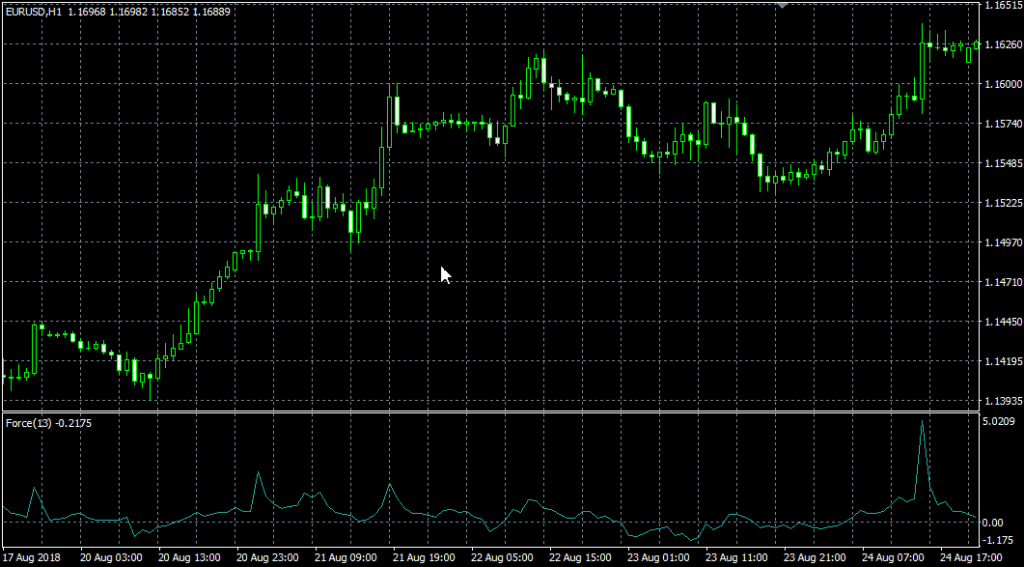There are four steps to follow to add new indicator to SQ X:

1. Adding new indicator building block
2. (Optional, recommended) Testing new indicator in SQ X vs data from MT
3. (Optional, recommended) Adding new signal block(s) based on the indicator
4. Adding translation of Indicator block into the language of target platform

## Step 1 – Creating new custom indicator in Code Editor

First step is to create our indicator in Code Editor. StrategyQuant internally uses Java programming language, and custom indicators must be programmed in Java.

You cannot take your MQL code and just copy & paste it to StrategyQuant, it wouldn’t work. You must rewrite the indicator into Java language.

First we have to open Code Editor: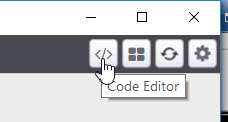In Code Editor you can create, edit and modify snippets, so we’ll create a new snippet for our new indicator.

Once in the Code Editor we click on Create new button on the top toolbar.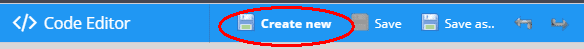This will open a dialogue, where we can name our new snippet and choose its type. We’ll name it “ForceIndex” and choose Indicator as a snippet type.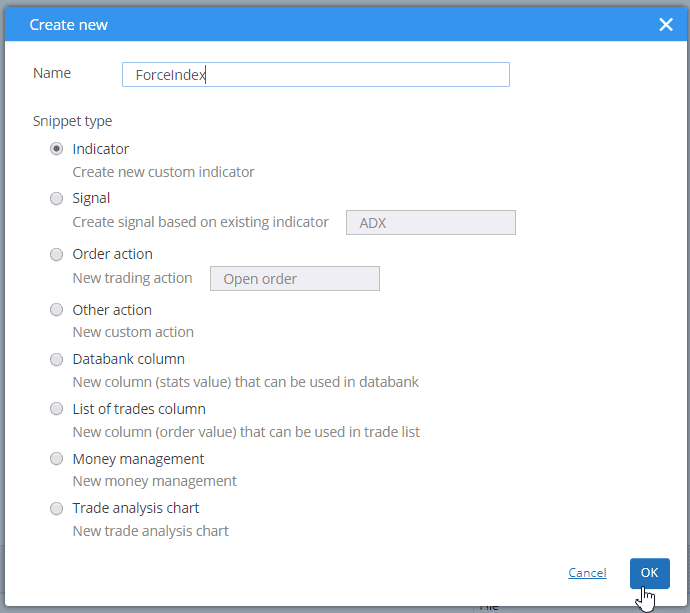After we click OK it will create a new folder ForceIndex under Snippets -> SQ -> Blocks -> Indicators and a new snippet file ForceIndex.java in this folder.

The convention in StrategyQuant is that every indicator is in its own folder – it is because later we might want to create signals for this indicator, and all the related snippets will be in the same folder.

This action created new file for our ForceIndex indicator and opened it in editor. You can see that the indicator is a class and it already has some structure.

Every indicator is extended from IndicatorBlock class and it must implement one method:

• OnBarUpdate() – method where indicator value is computed and stored to one of the output buffers. It Is called for every bar on chart.

When you’ll check the source code you should notice few things.

First, snippets in StrategyQuant use annotations a lot – @Parameter, @Output, @BuildingBlock – these annotations are used to declare a special property of a given variable or class – that the variable is a public parameter or output value, or that class is an indicator block.

Secondly, once you create and compile an indicator, you can call it from other methods or indicators by using Indicators.YourIndicator(YourIndicatorParameters). This is how our new indicator is called in OnBlockEvaluate() method.

We’ll go through the source code of the default indicator class created from template step by step:

```package SQ.Blocks.Indicators.ForceIndex;

import com.strategyquant.lib.*; import com.strategyquant.datalib.*; import com.strategyquant.tradinglib.*;

import SQ.Internal.IndicatorBlock;```

this is standard Java declaration of a package and imports of required classes – the ones we use in our own class.

```@BuildingBlock(name="(XXX) ForceIndex ", display="ForceIndex (#Period#)[#Shift#]", returnType = ReturnTypes.Price)
@Help("ForceIndex help text")```

Annotated definition of our indicator class, telling the system that it is a building block with given name.

• name field is what is displayed in UI when choosing building blocks.
• display field is what is displayed In Wizard with parameters. You can control which parameters are shown and in what place
• returnType is a type of indicator, it says what kind of value this indicator computes. It is used so that StrategyQuant knows which types should be compared with what. For example, it wouldn’t compare CCI (which returns Number) with Bolinger Bands (which returns Price).

There are basically three return types an indicator can have:

• Price – indicator computes price and is displayed on price chart – like Bollinger Bands, Moving Average, etc.
• Number – indicator computes number that is displayed on its own chart – like CCI, RSI, MACD, etc.
• PriceRange – indicator computes price range (difference f two prices) – like ATR

Other return types are used in another types of building blocks.

In our case ForceIndex IS NOT displayed on the price chart, so its return type is Number, not Price. We will change it in the next step.

```@Parameter     public DataSeries Input;

@Parameter(defaultValue = "10", isPeriod = true, minValue=1, maxValue=10000, step=1)     public int Period;

@Output     public DataSeries Value;```

what follows are indicator parameters. Every indicator can have multiple input parameters, the template creates just two of them as an example. Every parameter is annotated with @Parameter annotation, which could have several attributes.

The very first parameter is Input, it is the data series array from which the indicator is computed – it can be for example Open, High, Low or Close price.

Second parameter is Period, indicators usually have some period on which they are computed.

Third variable is Value, note that it has different annotation @Output. This means that this variable is not an indicator parameter but its output buffer. Indicators usually have just one output buffer, but they can have more – for example Bollinger band has Upper and Lower buffer.

There is one more hidden parameter Shift – it is by default in every indicator and it tells trading engine what value back it should look for. You generally don’t need to care about this parameter, it is used automatically.

Then there is a method:

`protected void OnBarUpdate() {...}`

This is the method where the indicator values are computed. It is called internally by SQ for every bar and it should compute indicator value for this bar and save it to the output buffer.

This is the source code of the standard indicator template. In the next step we’ll show the changes that must be made to implement our ForceIndex indicator.

## Step 2 – Modifying the generated default template and implementing the indicagtor

The indicator created in the step 1 is a standard indicator template, it doesn’t yet compute ForceIndex . To implement ForceIndex we must do a few things:

### Update its @BuildingBlocks annotation

We’ll update the annotation of this indicator as follows:

`@BuildingBlock(name="(FI) ForceIndex ", display="ForceIndex (#Nbr_Periods#, #Multiplier)[#Shift#]", returnType = ReturnTypes.Number)`

This is the simplest part. We’ll just update name of the indicator and add the actual new parameters (see below) to the display attribute.

We also changed returnType to Number, because this indicator computes numbers displayed on separate chart, it doesn’t output some price value.

### Define ForceIndex parameters

The first thing to do is little tricky – we must change the type of the default Input parameter. In the standard template it is defined as follows:

`@Parameter public DataSeries Input;`

it is parameter named Input, with type DataSeries. This is valid for a big portion of indicators that are computed from only one price. For example CCI, RSI, etc. indicators are usually computed from Close price. You can configure them to be computed from different price, for example from Open price, but still it is only one price array.

DataSeries type is an array of values type that holds values for Close prices, or for Open prices, or for Typical prices, etc.
However, if you’ll look at ForceIndex MQL source code, you’ll see that it computes its values from one of the price values and from Volume.

To be able to access multiple price arrays at once we’ll use different type for output:

`@Parameter public ChartData Chart;`

ChartData
type is an object that represents the whole chart – you’ll have access to Open, High, Low, Close, Volume prices in the given chart.

Quick note – choosing the right type for input data variable :
If indicator is computed from one price, use DataSeries.
If it is computed from multiple prices – for example High, Low, Close, etc. – use ChartData.

Then there is a Period parameter, we can leave it unchanged as well:

```@Parameter(defaultValue="10", isPeriod=true, minValue=2, maxValue=1000, step=1) public int Period;
```

Third parameter is moving average method:

`@Parameter(name="Method", defaultValue="0") @Editor(type=Editors.Selection, values="Simple=0,Exponential=1,Smoothed=2,Linear weighted=3") public int MAMethod;`

This is little bit more complex, because we define a selection list (combo box control) as edit control of this parameter. So when editing in Wizard, user will be able to choose from the predefined values.

Last parameter is applied price – price that should be used in the moving average computation:

`@Parameter(defaultValue="0") @Editor(type=Editors.Selection, values="Close=0,Open=1,High=2,Low=3,Median=4,Typical=5,Weighted=6") public int AppliedPrice;`

Note that we used some attributes in the @Parameter annotation:

• defaultValue sets default value of this parameter
• isPeriod=true tells SQ that this parameter is a period – they are handled in a special way, in the Builder configuration you can configure the minimum and maximum value for periods, so SQ needs to know which parameters are periods.
• minValue, maxValue, step are minimum and maximum values that will be generated for this parameter during the random generation process.

### Define ForceIndex outputs

ForceIndex indicator has only one output, so we can leave it also unchanged:

`@Output public DataSeries Value;`

The annotation @Output means that this is an output buffer for this indicator. Note that it is of DataSeries type, which means it is an array of double values.

### Implement OnBarUpdate() method

If you’ll look at ForceIndex MQL code you’ll see that it is quite simple, its MQL code is:

```int start() {
int nLimit;
int nCountedBars=IndicatorCounted();

//---- insufficient data
if(Bars<=ExtForcePeriod) return(0);

//---- last counted bar will be recounted
if(nCountedBars>ExtForcePeriod) nCountedBars--;
nLimit=Bars-nCountedBars;

//---- Force Index counted
for(int i=0; i<nLimit; i++)
ExtForceBuffer[i] = Volume[i] * (iMA(NULL,0,ExtForcePeriod,0,ExtForceMAMethod,ExtForceAppliedPrice,i) - iMA(NULL,0,ExtForcePeriod,0,ExtForceMAMethod,ExtForceAppliedPrice,i+1));

//---- done
return(0);
}```

By analyzing the algorithm, we can see that Force Index value at given candle is computed as:

ForceIndex[i] = Volume[i] *(MovAvg(Period, MAMethod, AppliedPrice)[i] – MovAvg(Period, MAMethod, AppliedPrice)
[i+1]

We can implement it in Java like this:

```protected void OnBarUpdate() throws TradingException {
DataSeries computedFrom = Chart.getSeries(AppliedPrice);

double indiValue = Chart.Volume.get(0) * (Indicators.MA(computedFrom, Period, MAMethod).Value.get(0) - Indicators.MA(computedFrom, Period, MAMethod).Value.get(1));

Value.set(0, indiValue);
}
```

In this case, indicator is quite simple and its computation requires virtually only one line in StrategyQuant.

We first get price to compute indicator from – by calling Chart.getSeries(AppliedPrice).
Then we compute new indicator value as a difference of average of current and previous price value multiplied by current volume.

This is all, now when we hit Compile and then restart SQ we will see our new ForceIndex indicator in Random Indicators Signals section.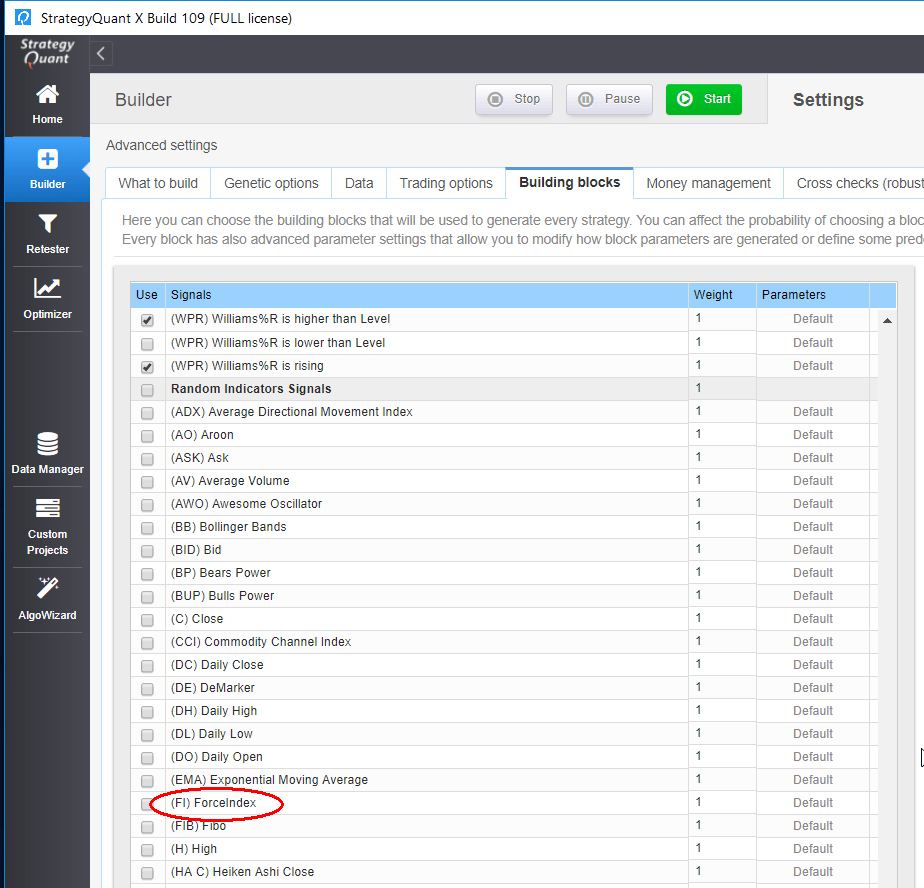Full source code of our new indicator:

```package SQ.Blocks.Indicators.ForceIndex;

import com.strategyquant.lib.*;
import com.strategyquant.datalib.*;

import SQ.Internal.IndicatorBlock;

/**
* Indicator name as it will be displayed in UI, and its return type.
* Possible return types:
* ReturnTypes.Price - indicator is drawn on the price chart, like SMA, Bollinger Bands etc.
* ReturnTypes.Price - indicator is drawn on separate chart, like CCI, RSI, MACD
* ReturnTypes.PriceRange - indicator is price range, like ATR.
*/
@BuildingBlock(name="(FI) ForceIndex ", display="ForceIndex (#Period#)[#Shift#]", returnType = ReturnTypes.Number)
@Help("ForceIndex help text")
public class ForceIndex extends IndicatorBlock {

@Parameter
public ChartData Chart;

@Parameter(defaultValue="10", isPeriod = true, minValue=2, maxValue=1000, step=1)
public int Period;

@Parameter(name="Method", defaultValue="0")
@Editor(type=Editors.Selection, values="Simple=0,Exponential=1,Smoothed=2,Linear weighted=3")
public int MAMethod;

@Parameter(defaultValue="0")
@Editor(type=Editors.Selection, values="Close=0,Open=1,High=2,Low=3,Median=4,Typical=5,Weighted=6")
public int AppliedPrice;

@Output
public DataSeries Value;

//------------------------------------------------------------------------
//------------------------------------------------------------------------
//------------------------------------------------------------------------

/**
* This method is called on every bar update and here the indicator value is computed.
*
* Unlike in MT4 you don't compute indicator values for multiple bars in a loop,
* you need to compute value only for the latest (current) bar.
* Trading engine will take care of calling this method for every bar in the chart.
*
* Actual bar for which the indicator value is computed is stored in CurrentBar variable.
* If 0, it means it is the very first bar of the chart.
*/
@Override
protected void OnBarUpdate() throws TradingException {
double indiValue;

indiValue = Chart.Volume.get(0) * (Indicators.MA(Chart.Close, Period, MAMethod).Value.get(0) - Indicators.MA(Chart.Close, Period, MAMethod).Value.get(1));

Value.set(0, indiValue);
}

}```